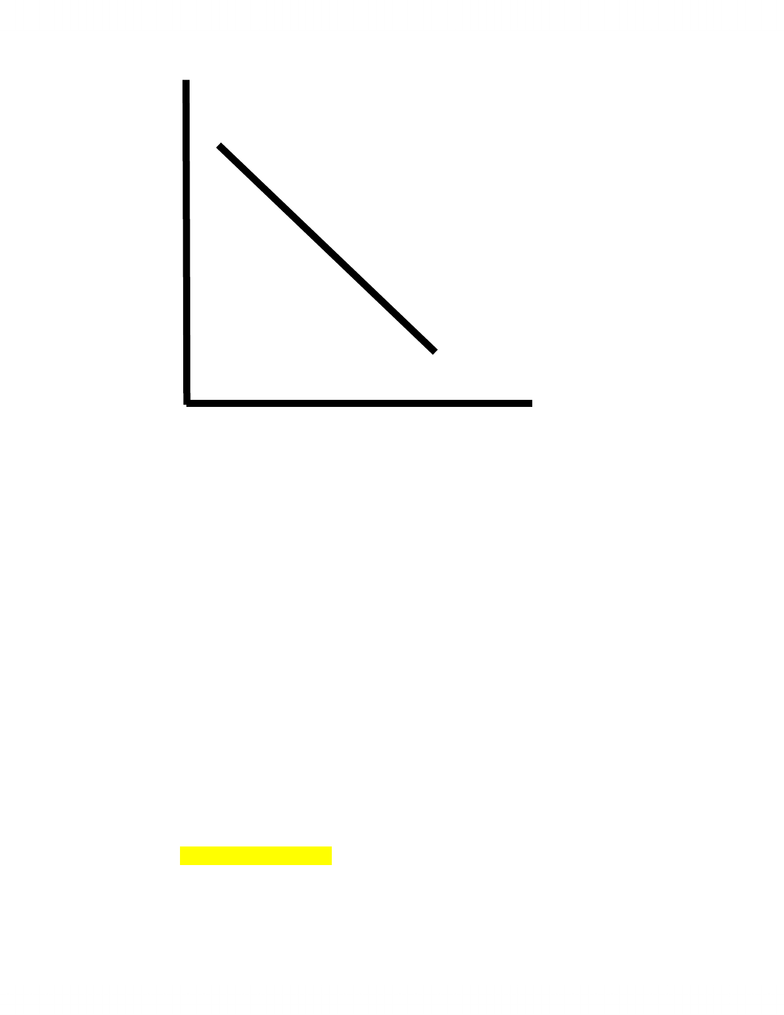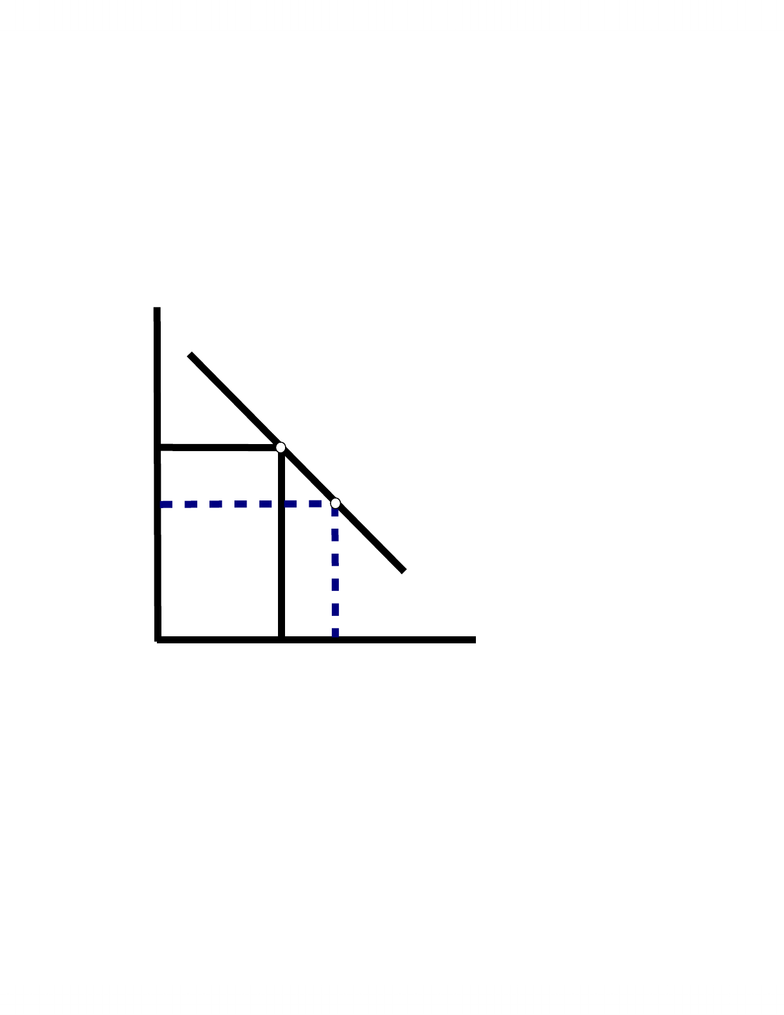48 views20 pages
School
University of Waterloo
Department
Economics
Course
ECON102Econ 102 Chapter 14 Notes Prof: Angela Trimarchi
Chapter 14
Aggregate Demand and Aggregate Supply
1Introduction
Recessions
http://en.wikipedia.org/wiki/Recession
Three Key Facts about Economic Fluctuations
Economic Fluctuations are Irregular & Unpredictable
Most Macroeconomic Quantities Fluctuate Together
As Output Falls, Unemployment Rises
Okun’s Law
Change in cyclical unemployment
-½ * (percent change in real GDP – 4%)
Exercise
oCalculate the change in cyclical unemployment when real GDP grows
at an average rate of 3%. 0.5
Aggregate Demand and Aggregate Supply Model
Used to explain short-run fluctuations in the economic activity around
its long-run trend
Aggregate Demand
Shows the relationship between the aggregate price level and the
total quantity of aggregate output demanded by households,
Aggregate Demand Curve
Graphs output (Y) and the price level (P)
Aggregate demand curve is downward sloping
Slope of the Aggregate Demand Curve
Recall the basic equation of national income accounting:
GDP = C + I + G + X – IM
Diagram 1: Aggregate Demand Curve
Unlock document

This preview shows pages 1-3 of the document.
Unlock all 20 pages and 3 million more documents.Econ 102 Chapter 14 Notes Prof: Angela Trimarchi
Aggregate demand curve slopes downward for reasons other than individual
demand curve:
Export-Import Effect
Interest Rate Effect on Investment
AD curve is drawn holding all other variables constant
i.e. the money supply and FXR
Export-Import Effect
An decrease in the Canadian price level (holding the foreign
exchange rate constant) makes Canadian goods cheaper than foreign
goods
This increases exports of Canadian goods and decreases
imports of foreign goods
Because exports increase net exports increase,
And so, the quantity of Canadian goods and services demanded
at a lower Canadian price level rises
Example:
Assume the nominal exchange rate is constant
Recall the definition for the real exchange rate:
e = enom * P/PF
Assume enom is 4 euros per dollar
P = \$200
P
Y
0
Unlock document

This preview shows pages 1-3 of the document.
Unlock all 20 pages and 3 million more documents.Econ 102 Chapter 14 Notes Prof: Angela Trimarchi
PF = 1600 euros
e is 4 * \$200/1600 = 0.50
Example:
Now assume the domestic price level falls to \$150
e is 4 * 150/1600 = 0.375
the real exchange rate falls
this means one unit of domestic goods buys fewer units of
foreign goods
so, the demand for domestic goods increases
Diagram 2: Export-Import Effect
Interest Rate Effect on Investment
A decrease in the price level decreases the transactions demand
for money
Therefore, the demand for money demand curve shifts left
When the money demand curve shifts , this decreases the
interest rate for a given money supply
A lower interest rate leads to an increase in investment
expenditures
Because I is a component of aggregate demand, there will be an
increase in the quantity of goods and services demanded
Diagram 3: A Decrease in the Money Demand
P
A
D
Y
0
P0
Y0
P1
Y1
Assume P falls
relative to PF
holding FXR
constant
Unlock document

This preview shows pages 1-3 of the document.
Unlock all 20 pages and 3 million more documents.